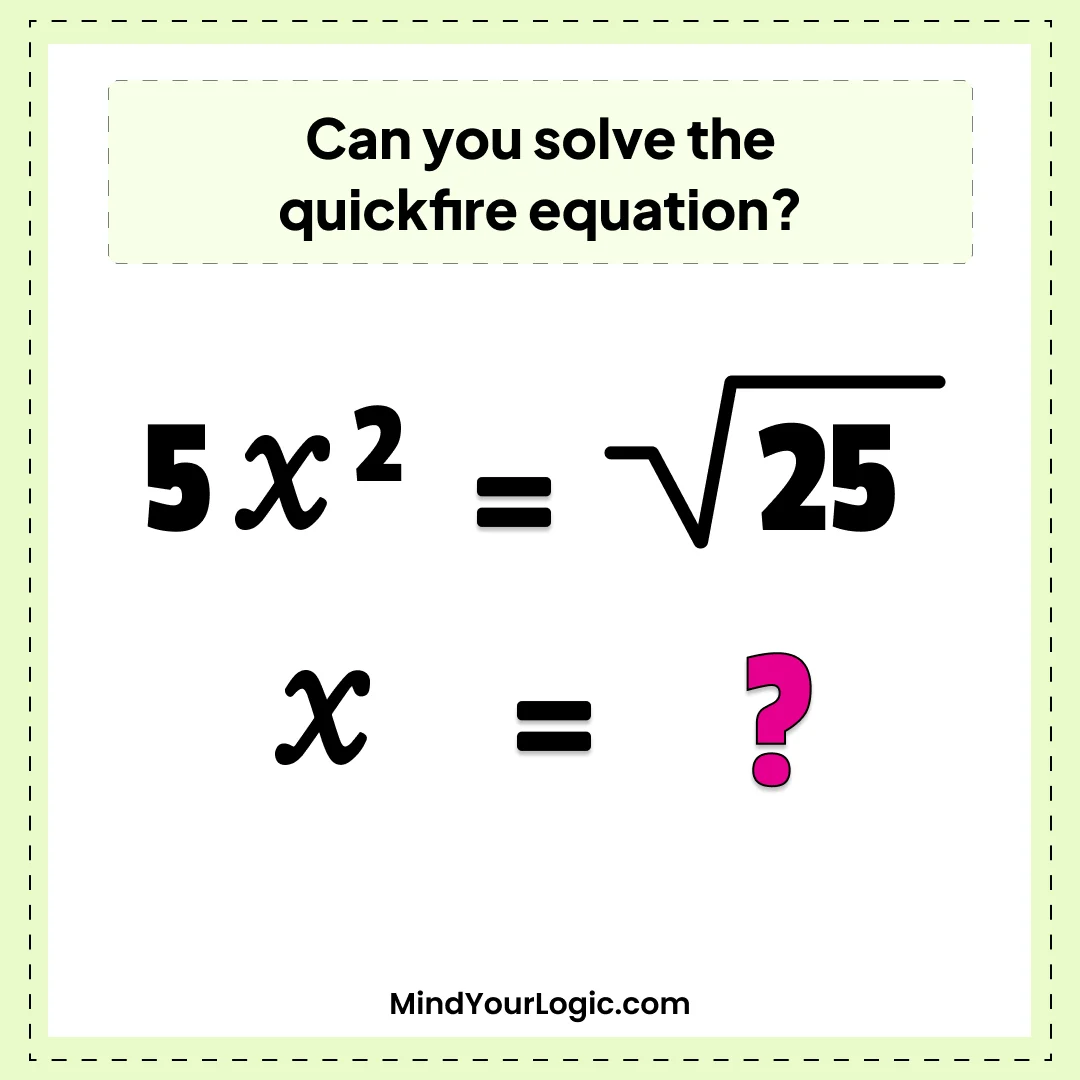# Quickfire equation? - Math Riddle

Can you solve the below quickfire equation?Explanation :

Ans  :  +  1
5x^2 = ?25
5x^2 = 5
X^2 = 5/5
X=?1 = +  1